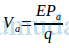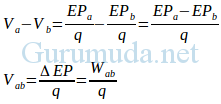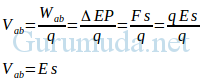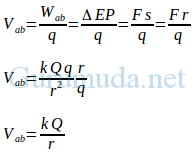# Electric Potential: Definition and Formula

Posted on

What is an electric potential? An Electric potential is graded as electrical level, and difference of two such levels, causes current to flow between them. When a body is charged, it can attract an oppositely charged body and can repulse a similar charged body. Electric potential is defined at a point in an electric field as the amount of work to be done to bring a unit positive electric charge from infinity to that point. When it’s at “a” point, q charge has an electric potential energy of the EPa, then the electric potential at the “a” point can be formulated:Note:

V: Electric Potential

EP: Electric Potential Energy

An Electric potential is not only at the “a”point but at all of the points in electric field. The “a”point is used as an example, the electric potential does not depend at the“q” charge. Electric potential energy and electric charge are scalar quantity so that the electric potential is also scalar quantity. International System of Units (SI) of electric potential energy is Joule and the International System of Units of electric charge is Coulomb, so the International System of Units, electric potential is expressed in units of joules per coulomb (I.e., volts), derived from the name of Italian scientist and inventor of the electric battery, Alesandro Volta (1745 – 1827).

# Electric Potential: Definition and Formula

An electric potential within “a” point e.g. electric potential at a point “a” i.e., Va, it’s not known as its value because it’s the change in electric potential. The change in electric potential can be known its value through calculation or measurement. Electric potential can change when “q” charge from 1 point to another points. Example, “q” charge moves from “a” point to “b” point so the change in electric potential is:Vab is an electric potential difference between 2 points in electric field, e.g. “a” point and “b” point. The Electric potential difference between “a” point and “b” point (Vab) is equal to the work done by electric force at electric charge when it moves from “a” point to “b” point, per unit charge (Wab/q). You need to know that the work done by electric force at “q” charge when it moves from “a” point to “b” point is the same with the change in electric potential energy “q” charge (ΔEP). Therefore in the above equation (ΔEP) can be replaced with Wab.

## Electric Potential in a Homogeneous Electric Field

An electric potential difference between two points in a homogeneous electric field, e.g. “a” point and “b” point can be calculated using equation:Note:

Vab: Electric potential difference between 2 points

E: Electric field

s: Distance between 2 points

## Electric Potential Evoked by a Single Charge

An Electric potential at a point due to a single charge that produces an electric field can be calculated using equation:Note:

Vab: Electric potential difference between 2 points

k: Constant Coulomb

Q: A single charge that creates an electric field

r: Distance between “Q” charge and a point where electric potential is calculated.

### Electric Potential  Conclusion

After going through the above portion of electric potential formula we can now establish an electric potential definition. I hope you enjoy when reading this article, thank you.